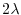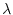Subsections

# Decoherence

libquantum provides an efficient model to simulate the effects of decoherence. The effects are simulated by a random rotation about the z axis, where the angle of the rotation is a normal distributed value with the variance.is the decoherence parameter, which depends on the experimental realization of the quantum computer. A list of values foris given in [DiVincenzo, 1995].

## quantum_set_decoherence

 extern void quantum_set_decoherence(float lambda);

This function sets the decoherence parameterwhich affects all following quantum operations.

## quantum_decohere

 extern void quantum_decohere(quantum_reg *reg);

Perform a decoherence step on a quantum register. Note that this function gets called automatically by all elementary gates provided by libquantum.